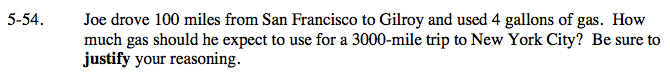Home > AC > Chapter 5 > Lesson 5.1.5 > Problem5-54

5-54.

Joe drove 100 miles from San Francisco to Gilroy and used 4 gallons of gas. How much gas should he expect to use for a 3000-mile trip to New York City? Be sure to justify your reasoning. Homework Help ✎Set up a proportion with the information in the problem.

Solve for x.

$\frac{\text{miles}}{\text{gallons}}=\frac{100}{4}=\frac{3000}{x}$

$4\left(\frac{100}{4}\right)=\left(\frac{3000}{x}\right)4$

$x(100)=\left(\frac{12000}{x}\right)x$

$100x = 12000$

x = 120 gallons